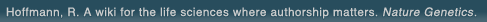# The world's first wiki where authorship really matters (Nature Genetics, 2008). Due credit and reputation for authors. Imagine a global collaborative knowledge base for original thoughts. Search thousands of articles and collaborate with scientists around the globe.

wikigene or wiki gene protein drug chemical gene disease author authorship tracking collaborative publishing evolutionary knowledge reputation system wiki2.0 global collaboration genes proteins drugs chemicals diseases compound
Hoffmann, R. A wiki for the life sciences where authorship matters. Nature Genetics (2008)# Molecular structure and conformational composition of 2-chloro-1-phenylethanone, ClH2C-C(=O)Ph, obtained using gas-phase electron-diffraction data and results from theoretical calculations.

The structure and conformation of 2-chloro-1-phenylethanone, ClH(2)C-C(=O)Ph (phenacyl chloride), have been determined by gas-phase electron diffraction (GED), augmented by results from ab initio molecular orbital calculations, employing the second-order MÃ¸ller-Plesset (MP2) level of theory and the 6-311+G(d) basis set. The molecules may exist as a mixture of different conformers with the C-Cl bond either syn (torsion angle phi = 0 degrees ) or gauche to the carbonyl bond. At 179 degrees C, the majority of the molecules (90 +/- 11%) have the gauche conformation (phi = 112(3) degrees). Torsion is also possible about the C-Ph single bond. Both experimental and theoretical data indicated, however, that the phenyl ring is coplanar or nearly coplanar with the carbonyl group. The results for the principal distances (r(g)) and angles (angle(alpha)) for the gauche conformer from a combined GED/ab initio study (with estimated 2sigma uncertainties) are the following: r(C-C)(phenyl) = 1.394(2) (average value) A, r(C(phenyl)-C(carbonyl)) = 1.484(5) A, r(C(carbonyl)-C(alkyl)) = 1.513(5) A, r(C-Cl) = 1.790(5) A, r(C=O) = 1.218(6) A, r(C-H)(phenyl) = 1.087(9) (average value) A, r(C-H)(alkyl) = 1.090(9) A (average value), angle C(phenyl)-C=O = 119.5(9) degrees, angle C(phenyl)-C(carbonyl)-C(alkyl) = 119.2(10) degrees, angle C-C-Cl = 109.8(12) degrees, angle C(2)-C(1)-C(carbonyl) = 122.8(15) degrees, angle C-C(alkyl)-H = 111.2 degrees (ab initio value).

## References

1. Molecular structure and conformational composition of 2-chloro-1-phenylethanone, ClH2C-C(=O)Ph, obtained using gas-phase electron-diffraction data and results from theoretical calculations. Aarset, K., Hagen, K. The journal of physical chemistry. A, Molecules, spectroscopy, kinetics, environment & general theory. (2005) [Pubmed]